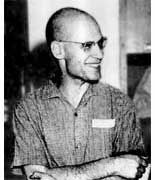We have seen that a non-commutative $l$-point is an
algebra$P=S_1 \\oplus … \\oplus S_k$with each $S_i$ a simple
finite dimensional $l$-algebra with center $L_i$ which is a separable
extension of $l$. The centers of these non-commutative points (that is
the algebras $L_1 \\oplus … \\oplus L_k$) are the open sets of a
Grothendieck-topology on
$l$. To define it properly, let $L$ be the separable closure of $l$
and let $G=Gal(L/l)$ be the so called absolute
Galois group. Consider the
category with objects the finite $G$-sets, that is : finite
sets with an action of $G$, and with morphisms the $G$-equivariant
set-maps, that is: maps respecting the group action. For each object
$V$ we call a finite collection of morphisms $Vi \\mapsto V$ a
cover of $V$ if the images of the finite number of $Vi$ is all
of $V$. Let $Cov$ be the set of all covers of finite $G$-sets, then
this is an example of a Grothendieck-topology as it satisfies
the following three conditions :

(GT1) : If
$W \\mapsto V$ is an isomorphism of $G$-sets, then $\\{ W \\mapsto V \\}$ is an element of $Cov$.

(GT2) : If $\\{ Vi \\mapsto V \\}$ is in $Cov$ and if for every i also $\\{ Wij \\mapsto Vi \\}$
is in $Cov$, then the collection $\\{ Wij \\mapsto V \\}$ is in
$Cov$.

(GT3) : If $\\{ fi : Vi \\mapsto V \\}$ is in $Cov$
and $g : W \\mapsto V$ is a $G$-morphism, then the fibered
products
$Vi x_V W = \\{ (vi,w) in Vi x W : fi(vi)=g(w) \\}$is
again a $G$-set and the collection $\\{ Vi x_V W \\mapsto V \\}$
is in $Cov$.

Now, finite $G$-sets are just
commutative separable $l$-algebras (that is,
commutative $l$-points). To see this, decompose a
finite $G$-set into its finitely many orbits $Oj$ and let $Hj$ be the
stabilizer subgroup of an element in $Oj$, then $Hj$ is of finite
index in $G$ and the fixed field $L^Hj$ is a finite dimensional
separable field extension of $l$. So, a finite $G$-set $V$
corresponds uniquely to a separable $l$-algebra $S(V)$. Moreover, a
finite cover $\\{ W \\mapsto V \\}$ is the same thing as saying
that $S(W)$ is a commutative separable $S(V)$-algebra. Thus,
the Grothendieck topology of finite $G$-sets and their covers
is anti-equivalent to the category of commutative separable
$l$-algebras and their separable commutative extensions.

This raises the natural question : what happens if we extend the
category to all separable $l$-algebras, that is, the category of
non-commutative $l$-points, do we still obtain something like a
Grothendieck topology? Or do we get something like a
non-commutative Grothendieck topology as defined by Fred Van
Oystaeyen (essentially replacing the axiom (GT 3) by a left and right
version). And if so, what are the non-commutative covers?
Clearly, if $S(V)$ is a commutative separable $l$-algebras, we expect
these non-commutative covers to be the set of all separable
$S(V)$-algebras, but what are they if $S$ is itself non-commutative,
that is, if $S$ is a non-commutative $l$-point?

Published in featured